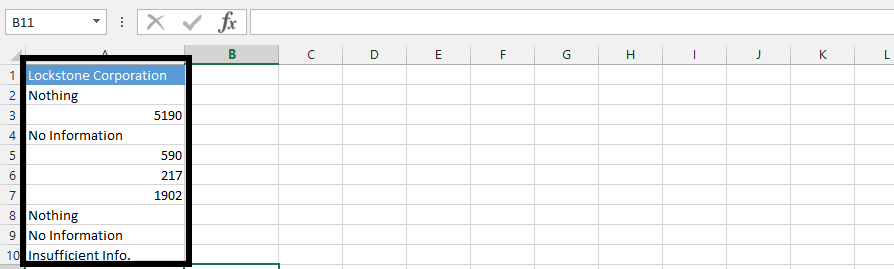### How To Return Many Items For A Single Lookup Value

There are different ways to return many items for a single lookup value in Excel. But in this tutorial article, we are going to use different functions in one single formula to find many items. But for the successfulness of this, the first thing you need is data that looks like this:Note: You could see that there are multiple factors that shows in the data as number.Click on an empty cell (1), and type in =COUNT(A4:A10) in the formula box (2).Click on an empty cell (1), and type

=IF(ROWS(C\$2:C2)<=\$B\$2,INDEX(\$A\$2:\$A\$10,SMALL(IF(ISNUMBER(\$A\$2:\$A\$10),ROW(\$A\$2:\$A\$10)-ROW(\$A\$2)+1), ROWS(C\$2:C2))),””) (2),

and then press F2.

Now hold CTRL + SHIFT simultaneously, and press enter.Note: We used the small box on the right lower corner of the cell to drag down the result that is showing on the picture above.

## Template

```Further reading: